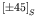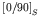Computational & Technology Resources an online resource for computational,engineering & technology publications not logged in - login Civil-Comp ProceedingsISSN 1759-3433 CCP: 83PROCEEDINGS OF THE EIGHTH INTERNATIONAL CONFERENCE ON COMPUTATIONAL STRUCTURES TECHNOLOGY Edited by: B.H.V. Topping, G. Montero and R. Montenegro Paper 275Analysis of the Free-Edge Effect in Piezoelectric Laminated Plates by the Scaled Boundary Finite-Element Method J. Artel and W. BeckerDepartment of Mechanical Engineering, Darmstadt University of Technology, Germany doi:10.4203/ccp.83.275 Full Bibliographic Reference for this paper J. Artel, W. Becker, "Analysis of the Free-Edge Effect in Piezoelectric Laminated Plates by the Scaled Boundary Finite-Element Method", in B.H.V. Topping, G. Montero, R. Montenegro, (Editors), "Proceedings of the Eighth International Conference on Computational Structures Technology", Civil-Comp Press, Stirlingshire, UK, Paper 275, 2006. doi:10.4203/ccp.83.275 Keywords: scaled boundary finite-element method, free-edge effect, piezoelectricity. Summary The paper deals with the analysis of the free-edge effect in laminated plates composed of piezoelectric materials by using the scaled boundary finite-element method. This novel method combines the advantages of the finite element method and the boundary element method as the method is finite element based although a discretization of the boundary of the considered domain is sufficient. Applying the scaled boundary finite-element method to the analysis of free-edge effect situations necessitates the implementation of kinematic coupling equations, which is shown in detail. Finally, a symmetric cross-ply laminate under uniform strain is investigated exemplarily to show the efficiency of the new method. The occurrence of interlaminar stresses in the vicinity of free edges in laminated plates is of significant practical importance and is the aim of many analytical and numerical investigations within many years [1,2]. Generally, some interlaminar stress components become singular within the interface between two dissimilar adjacent layers. This leads to the assumption, that laminated plates consisting out of piezoelectric materials behave similar and that some components of the electric field strength vector also may become singular. Davi and Milazzo investigated asymmetric angle-ply laminate under several loading conditions by using the boundary element method . Within this paper, the investigation of this effect is performed by using the scaled boundary finite-element method and the results are compared with those generated by a finite element analysis. Both methods use full electromechanical coupling to simulate the piezoelectric material behaviour. The scaled boundary finite-element method is a novel semi-analytical method, which has been developed by Wolf and Song . The method is based on the introduction of a local coordinate system, where the governing equations are employed in the weak form in two local directions and in the strong form in the so called scaling direction. The solution is achieved by approximating the weak form by a finite element approach and by solving the strong form analytically as it is given by a system of ordinary differential equations. Although a discretization of the boundary of the domain is sufficient, the method is finite element based and no fundamental solution is required within the analysis procedure. The method is applied to the analysis of the free-edge effect in piezoelectric laminated plates. A laminated plate is considered, which is extended to infinity in the scaling direction perpendicular to the free edge and in the direction parallel to the free edge. Within the scaled boundary finite-element method the corresponding model is chosen to be represented by a slat in the scaling direction, where the free edge is discretized with elements. The loading is applied by a given strain field, which is taken into account by corresponding kinematic coupling equations. In order to fulfill the condition of an infinite extended laminate in the direction parallel to the free edge, special degrees of freedom have to be introduced on the side-faces of the slat to hold this condition for the electric potential. The coupling of degrees of freedom is taken into account by a general linear relation between dependent and independent coupled degrees of freedom and a constant vector, which can be considered as a given displacement field. Generally, the coupling equations also have to be fulfilled in the scaling direction, which consequently leads to an extended formulation of the method. The implementation of the kinematic coupling equations within the method is presented. Furthermore, degrees of freedom are introduced, that are no functions of the scaling coordinate, which is necessary for the coupling of the electric potential. As a special problem, rigid body modes are handled as they occur in any free-edge analysis using the scaled boundary finite-element method. The condition of zero resultant forces in the direction of the rigid body modes can be fulfilled in order to obtain a valid solution. By using the theory of generalized inverse matrices, the solution procedure is completed. Asymmetric cross-ply laminate is analyzed under a given uniaxial stress field parallel to the free edge and the interlaminar stresses and electric field strengths are investigated. It is shown, that some electric field strength components become singular in the vicinity of the free edge. The comparison of the results generated by the scaled boundary finite-element method and the finite element methods shows very good accordance, but the scaled boundary finite-element method requires considerably less numerical effort. References 1 R.B. Pipes, N.J. Pagano, "Interlaminar Stresses in Composite Laminates Under Uniform Axial Extension", Journal of Composite Materials 4, 538-548, 1970.doi:10.1177/002199837000400409¬¬ 2 C. Mittelstedt, W. Becker, "Interlaminar Stress Concentrations in Layered Structures, Part I: A Selective Literature Survey on the Free-Edge Effect since 1967", Journal of Composite Materials, Vol. 38, No. 12, 1037-1062, 2004. doi:10.1177/0021998304040566 3 G. Davi and A. Milazzo, "Stresses and Electric Fields in Piezoelectric Composite Laminates", Electric Journal of Boundary Elements, Vol. BETEQ 2001, No. 1, 43-50, 2002. 4 J.P. Wolf, C. Song, "Finite-element modelling of unbounded media", Wiley & Sons Ltd, Chichester, 1996. purchase the full-text of this paper (price £20) Back to top ©Civil-Comp Limited 2022 - terms & conditions